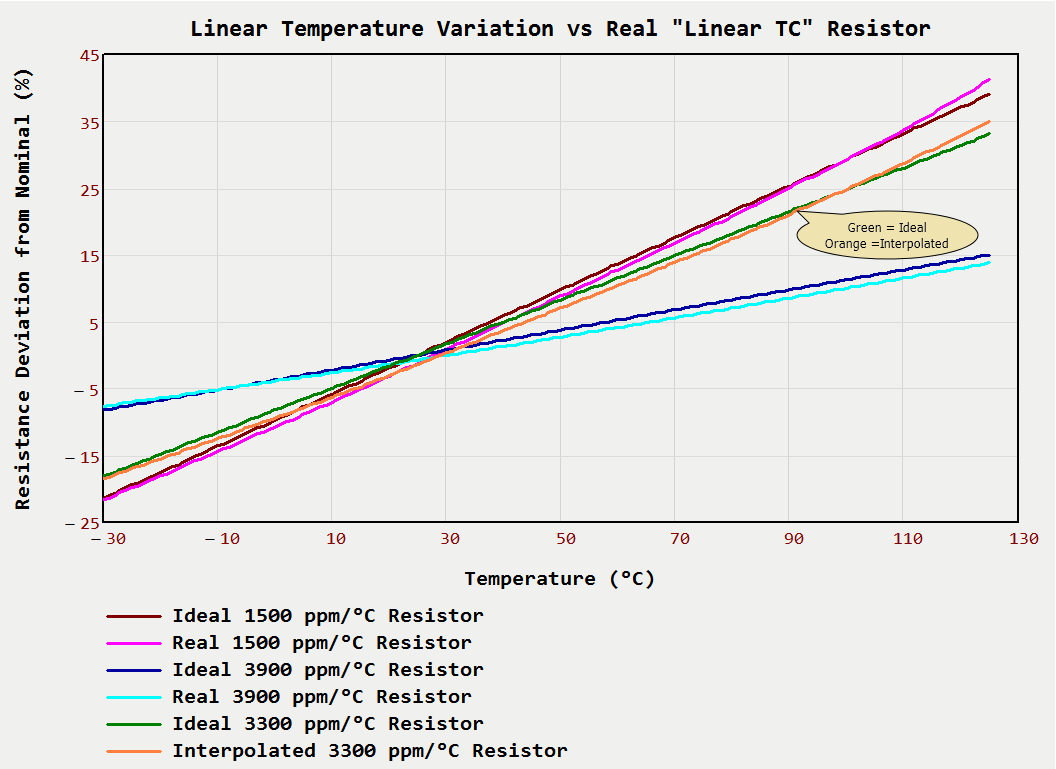# Linear Temperature Coefficient Resistor Nonlinearity

Quote of the Day

Having proper motivation and honesty are the keys to overcoming fear or anxiety. Fearless and honest self-appraisal can be a powerful weapon against self-doubt or low self-confidence.

— Dalai Lama

## IntroductionFigure 1: Resistance Variation with Temperature of a Real "Linear" Temperature Coefficient Resistor.

Our products contain many analog circuits, and these circuits often require temperature compensation in order to meet their requirements across the product's entire temperature range. To perform this compensation, we often use resistors with a specified Temperature Coefficients of Resistance (TCR). A vendor recently stopped manufacturing one of the resistors we use for temperature compensation, and we needed to find a substitute. While searching for a substitute resistor, I needed to understand just how linear the approved resistor's temperature variation is so I can find an appropriate substitute.

Unfortunately, the original designer (gone for over ten years) used a TCR for which the vendor supported but had not published resistance versus temperature curves. The vendor did provide resistance curves for three similar temperature coefficients, which allows me to use interpolation to estimate the curves for the value we are using. Of course, I am requesting that the vendor send me the correct curve. In the meantime, I am just going to interpolate between the specified curves for a preliminary result.

Figure 1 shows my plot of:

• resistance versus temperature curves for two different TCRs (3900 ppm/°C and 1500 ppm/°C)
• my interpolated resistance versus temperature curve for a TCR of 3300 ppm/°C.

You can see that there is a small error between the linear ideal and reality. I need to find a substitute part with a similar level of nonlinearity. This post is about viewing the nonlinearity – selecting the substitute part is another matter.

## Background

### Model for Resistance Variation with Temperature

Equation 1 shows the formula for the variation in resistance for a linear temperature coefficient (i.e. constant TCR) resistor.

 Eq. 1$\displaystyle R\left( T \right)={{R}_{{ref}}}\cdot \left( {1+\alpha \cdot \left( {T-{{T}_{{ref}}}} \right)} \right)$

where

• R(T) is the resistor's resistance at a temperature of T.
• Rref is the resistor's resistance at a temperature of Tref.
• Tref is temperature at which the resistor's nominal resistance is specified.
• α is the resistor's TCR.

In Figure 1, I used Equation 1 as my ideal temperature function.

### Approach

My analysis approach is simple:

• Digitize the vendor's three resistance plots (TCRs = 1550, 2700, and 3900 ppm/°C) using Dagra.
• Perform a two-dimensional interpolation to estimate the response for a TCR = 3300 ppm/°C.
• Plot  both the ideal and the interpolated responses for a visual comparison of the level of nonlinearity.

## Analysis

The analysis was performed in Mathcad and is best viewed either in the Mathcad source or viewing the PDF, both of which are included here.

## Conclusion

I am surprised at the typical level of nonlinearity that I am seeing for these resistors. I have always thought that the temperature coefficient for these devices would be a constant, but there is a significant nonlinear component.

This entry was posted in Electronics. Bookmark the permalink.

### One Response to Linear Temperature Coefficient Resistor Nonlinearity

1.Louis brown says:

We have an RF power amp that needs temperature compensation on both the gate bias and ALC detector diodes. I ended up using two thermistors and a few fixed resistor. Then brute force compute all the combinations to reduce the MSE to fit a desired curve. 20 years ago it took 1 hour to compute, now it takes 1 second.